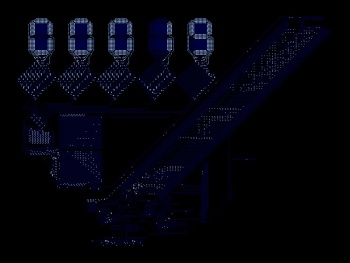## Electronic circuit simulation

General FreeBASIC programming questions.
Posts: 1461
Joined: May 24, 2007 22:10
Location: The Netherlands

### Electronic circuit simulation

Hello all (electronics enthusiasts), I am trying to simulate a relative simple circuit (see drawing in code). The result seems plausible. Anyone who wants to check the calculation steps?

Code: Select all

`' Circuit:''    +---(L1)---+---(L2)---+'    |          |          |'    |          |          |'   (Us)       (C1)       (R1)'    |          |          |'    |          |          |'    +----------+----------+'' Inductor:  U = L * dI / dt  or  dI = U * dt / L'' Capacitor: I = C * dU / dt  or  dU = I * dt / C'' Resistor: U = I * R'known/set valuesdim as double Us = 200 'V (power supply)dim as double L1 = 320 * 1e-6 'Hdim as double L2 = 100 * 1e-6 'Hdim as double C1 = 10 * 1e-6 'Fdim as double R1 = 1.0 'Ohm'to be calculated / intermediate valuesdim as double U_L1, U_C1, U_L2, U_R1 'Vdim as double I_L1, I_C1, I_L2, I_R1 'Adim as double dU_L1, dU_C1, dU_L2, dU_R1 'Udim as double dI_L1, dI_C1, dI_L2, dI_R1 'A'simulation time settingsdim as double t = 0, tEnd = 2e-3 'sdim as double dt = (tEnd - t) / 10000 'sconst W = 800, H = 600screenres W, H, 32width W \ 8, H \ 16'iterate over timewhile t < tEnd   'step 1, determine I_L1   U_L1 = Us - U_C1   dI_L1 = U_L1 * dt / L1   I_L1 += dI_L1   'step 2, determine U_C1   I_C1 = I_L1 - I_L2   dU_C1 = I_C1 * dt / C1   U_C1 += dU_C1   'step 3, determine U_R1   I_R1 = I_L2   U_R1 = I_R1 * R1   'step 4, determine I_L2   U_L2 = U_C1 - U_R1   dI_L2 = U_L2 * dt / L2   I_L2 += dI_L2   'plot at top: Voltages   pset((t / tEnd) * (W - 20) + 10, H * 0.4 - Us), rgb(0, 0, 255)    pset((t / tEnd) * (W - 20) + 10, H * 0.4 - U_C1), rgb(0, 255, 0)    pset((t / tEnd) * (W - 20) + 10, H * 0.4 - U_R1), rgb(255, 0, 0)   'plot at bottom: Currents   pset((t / tEnd) * (W - 20) + 10, H * 0.9 - I_L1), rgb(0, 0, 255)    pset((t / tEnd) * (W - 20) + 10, H * 0.9 - I_C1), rgb(0, 255, 0)    pset((t / tEnd) * (W - 20) + 10, H * 0.9 - I_L2), rgb(255, 0, 0)   'update time   t += dtwend'display what is whatdraw string (10, 10), "Us (blue), U_C1 (green), U_R1 (red)"draw string (10, H - 30), "I_L1 (blue), I_C1 (green), I_L2 (red)"'show I_R1 end valuedraw string (W \ 2, H \ 2), "I_R1 [A] = " + str(I_R1)'wait until key presswhile inkey = "" : wend`
paul doe
Posts: 919
Joined: Jul 25, 2017 17:22
Location: Argentina

### Re: Electronic circuit simulation

One amazing cellular automata that simulates electronic circuits is Wireworld. Here's my implementation in FreeBasic to play with:

https://github.com/glasyalabolas/fb-wireworldIncluded is the Wireworld computer that computes prime numbers. It may take a while to output one, but that depends mostly on your hardware. Electronics is quite a fascinating topic, indeed ;)
BasicCoder2
Posts: 3397
Joined: Jan 01, 2009 7:03

### Re: Electronic circuit simulation

deleted.
Last edited by BasicCoder2 on Apr 17, 2019 13:09, edited 1 time in total.
fxm
Posts: 9126
Joined: Apr 22, 2009 12:46
Location: Paris suburbs, FRANCE

### Re: Electronic circuit simulation

badidea wrote:The result seems plausible. Anyone who wants to check the calculation steps?
It seems to be good.
Posts: 1461
Joined: May 24, 2007 22:10
Location: The Netherlands

### Re: Electronic circuit simulation

fxm wrote:
badidea wrote:The result seems plausible. Anyone who wants to check the calculation steps?
It seems to be good.

Thanks, I checked the result with LTspice. Looked the same.
I am going to add more stuff (that I don't know how to do in LTspice).